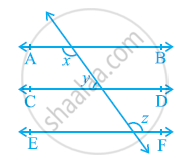# In the given figure, if AB || CD, CD || EF and y: z = 3: 7, find x. - Mathematics

In the given figure, if AB || CD, CD || EF and y: z = 3: 7, find x.#### Solution

It is given that AB || CD and CD || EF

∴ AB || CD || EF (Lines parallel to the same line are parallel to each other)

It can be observed that

x = z (Alternate interior angles)             ...........(1)

It is given that yz = 3: 7

Let the common ratio between y and z be a.

∴ y = 3a and z = 7a

Also, x + y = 180º (Co-interior angles on the same side of the transversal)

y = 180º                [Using equation (1)]

7a + 3a = 180º

10a = 180º

a = 18º

∴ x = 7a = 7 × 18º = 126º

Concept: Lines Parallel to the Same Line
Is there an error in this question or solution?
Chapter 6: Lines and Angles - Exercise 6.2 [Page 104]

#### APPEARS IN

NCERT Class 9 Maths
Chapter 6 Lines and Angles
Exercise 6.2 | Q 2 | Page 104

Share